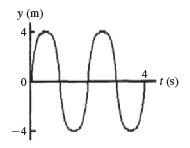# Problem: What is the frequency of the wave shown in the figure?a. 2 Hz. b. 4 Hz. c. 1 Hz. d. It cannot be determined from the given information. e. 0.5 Hz.

###### FREE Expert Solution

Frequency:

$\overline{){\mathbf{f}}{\mathbf{=}}\frac{\mathbf{1}}{\mathbf{T}}}$###### Problem Details

What is the frequency of the wave shown in the figure?a. 2 Hz.

b. 4 Hz.

c. 1 Hz.

d. It cannot be determined from the given information.

e. 0.5 Hz.